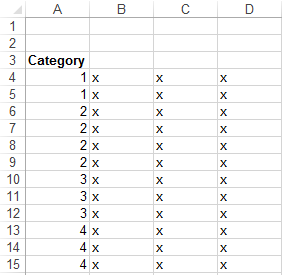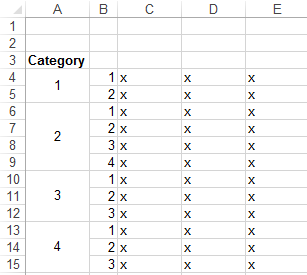# Merge and Label cells if same value

How do I merge cells of same value then label each row of the merged cell by a serial number?
Assuming I have this:and I am trying to achieve this:I found a way to merge the cells if they are of the same value
``````For i = 4 To Range("A" & Rows.Count).End(xlUp).Row
If Cells(i, 1) <> "" Then
If Cells(i, 1) = Cells(i - 1, 1) Then
Range(Cells(i, 1), Cells(i - 1, 1)).Merge
End If
End If
``````

Any suggestions on how I can proceed with the labelling? Maybe a "do while", but I'm not sure how to make sure it restarts from 1 if the adjacent cell is not merged.